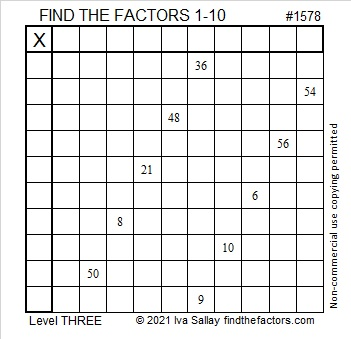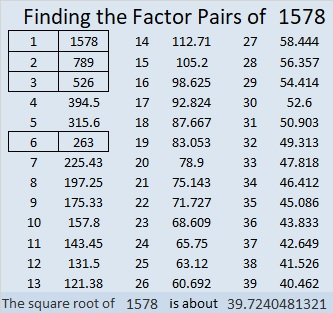# 1578 The Logic Needed to Solve This Puzzle is Straight Forward

### Today’s Puzzle:

What is the only common factor of 36 and 9 that will use only numbers from 1 to 10 in the first column? Answer that question, put the factors in their appropriate cells, and then go straight down the puzzle row by row, filling in factors as you go. In no time at all, you will have solved this level 3 puzzle!### Factors of 1578:

1 + 5 + 7 + 8 = 21, a number divisible by 3, so even number 1578 is divisible by 6.

• 1578 is a composite number.
• Prime factorization: 1578 = 2 × 3 × 263.
• 1578 has no exponents greater than 1 in its prime factorization, so √1578 cannot be simplified.
• The exponents in the prime factorization are 1, 1, and 1. Adding one to each exponent and multiplying we get (1 + 1)(1 + 1)(1 + 1) = 2 × 2 × 2 = 8. Therefore 1578 has exactly 8 factors.
• The factors of 1578 are outlined with their factor pair partners in the graphic below.### More about the Number 1578:

1578 is a leg in two Pythagorean triples:
1578-622520-622522, calculated from 2(789)(1), 789² – 1², 789² – 1², and
1578-69160-69178, calculated from 2(263)(3), 263² – 3², 263² – 3².